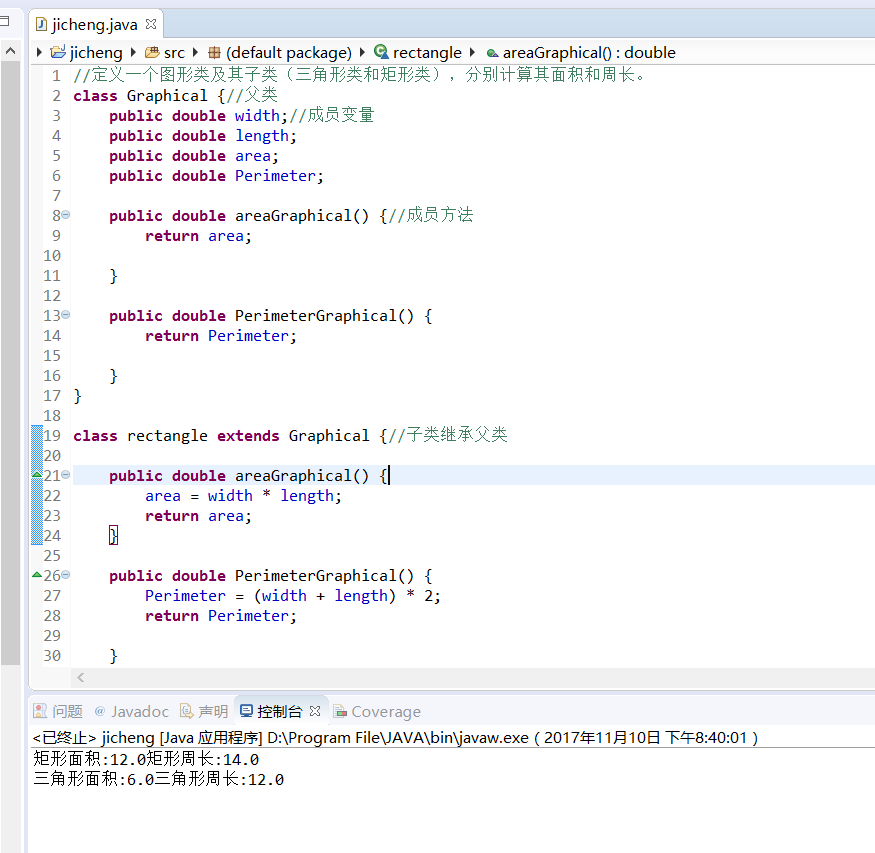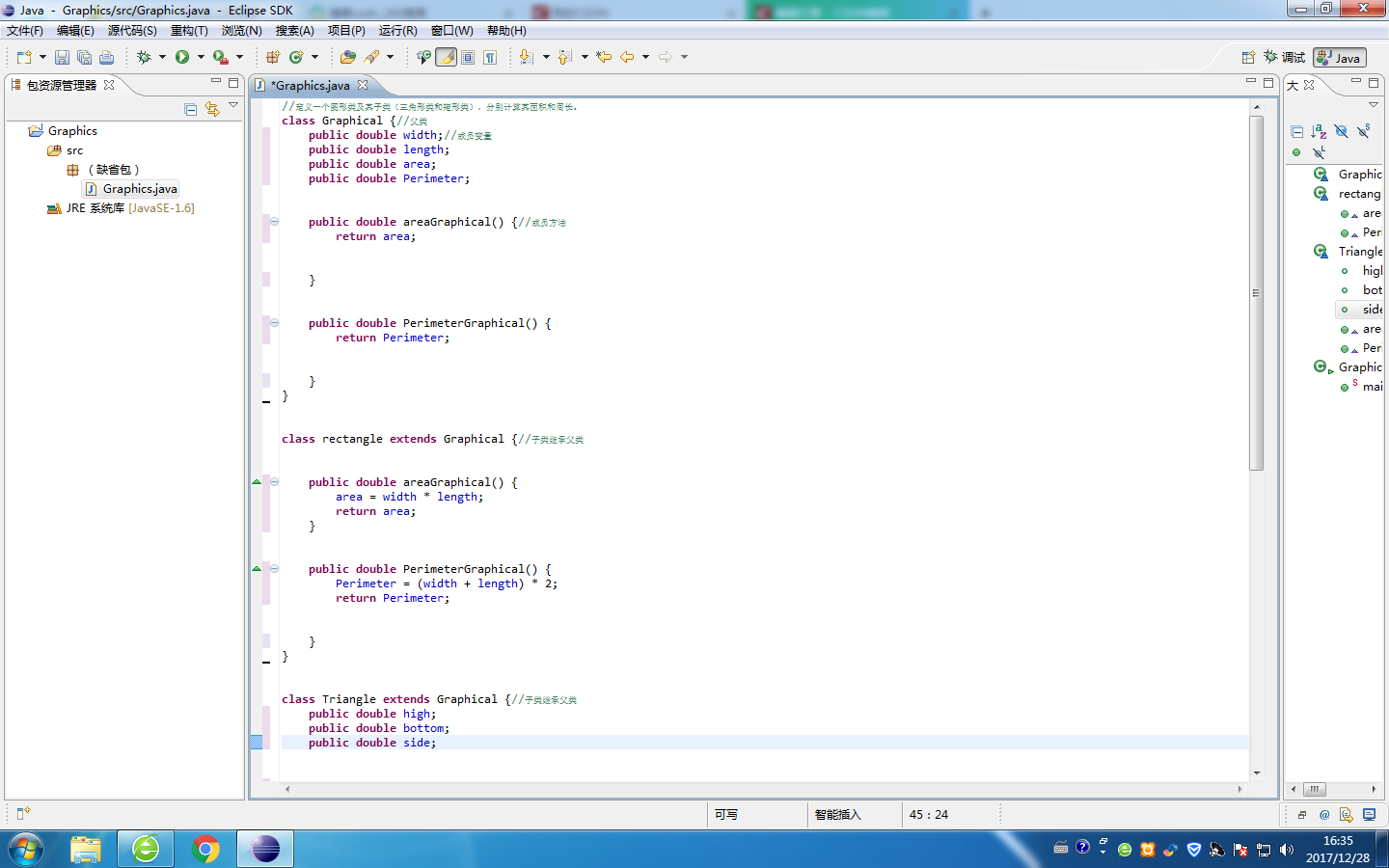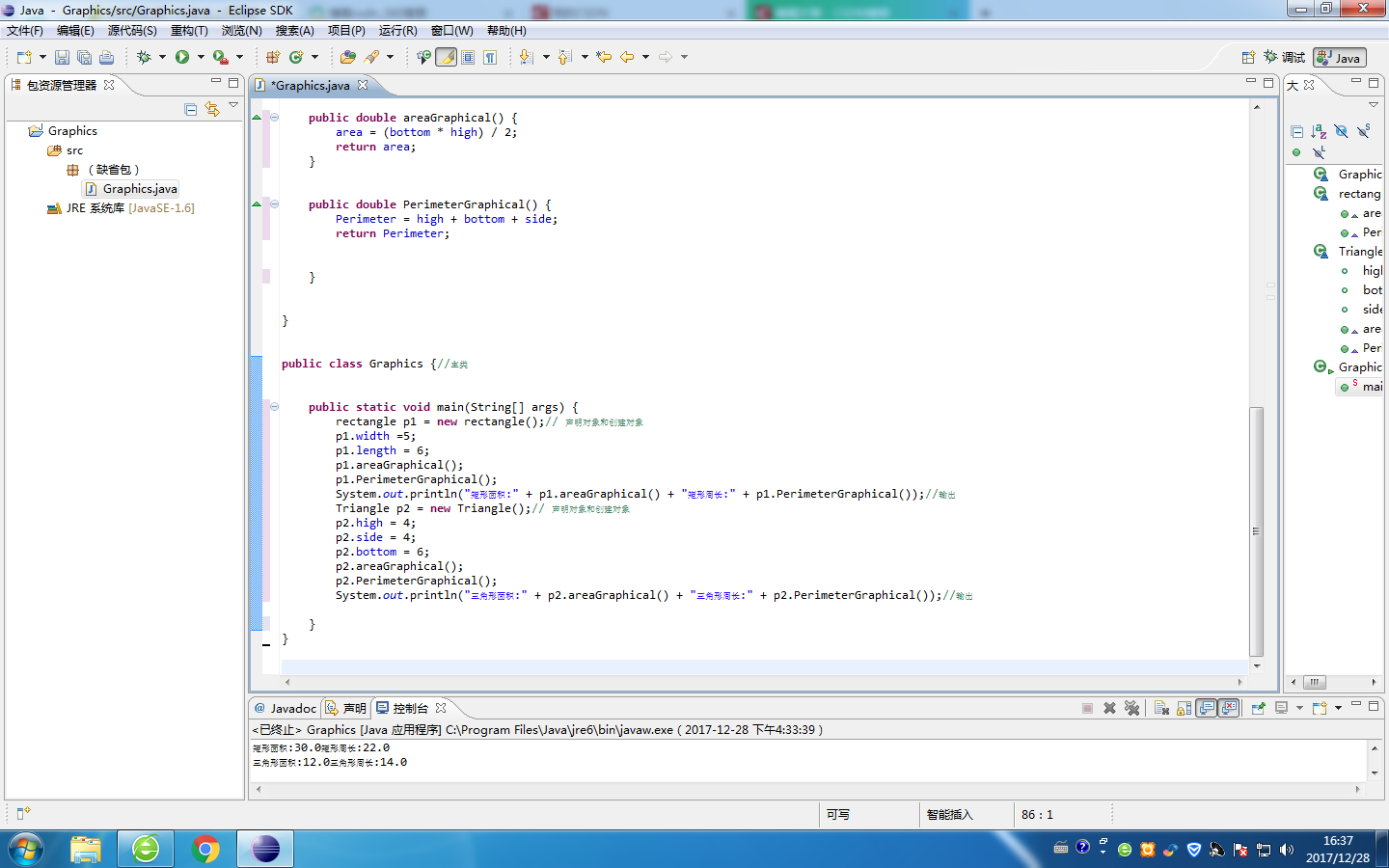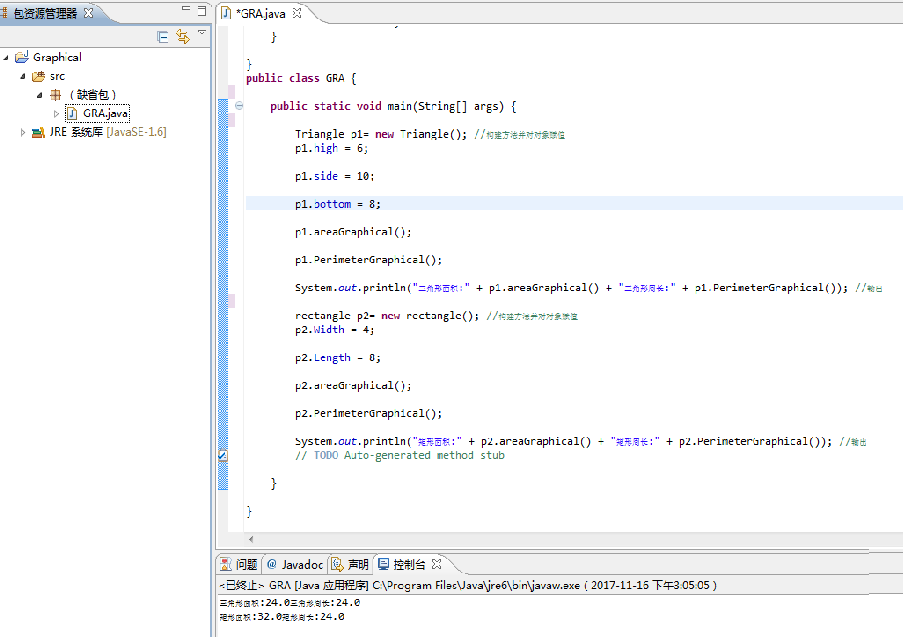• 定义抽象类Shape，圆形Circle、长方形Square、Rectangle为子类。 Shape类有一个数据成员（double型），定义带参构造方法、抽象方法calArea()(返回值类型：double)在Circle、Square两个类中，实现calArea()方法。在...
定义抽象类Shape，圆形Circle、长方形Square、Rectangle为子类。
Shape类有一个数据成员（double型），定义带参构造方法、抽象方法calArea()(返回值类型：double)在Circle、Square两个类中，实现calArea()方法。在Rectangle类中，增加一个数据成员（double型），实现calArea()方法。编写测试类：定义一个Shape类引用shape，分别指向一个Circle类、Square类、Rectangle类对象，利用多态，计算各平面图形的面积并输出。（结果保留三位小数）要求：1.数据成员全部为私有成员；2.数据从键盘输入，调用带参构造方法创建子类对象。提示：使用Math类的PI
import java.util.Scanner;
public class ShapeDemo {
public static void main(String[] args) {
Scanner in=new Scanner(System.in);
double a,b,c,d;
a=in.nextDouble();
b=in.nextDouble();
c=in.nextDouble();
d=in.nextDouble();
Shape sh=new Circle(a);
System.out.printf("圆的面积：%.3f",sh.calArea());
System.out.println();
sh=new Square(b);
System.out.printf("正方形的面积：%.3f",sh.calArea());
System.out.println();
sh=new Rectangle(c,d);
System.out.printf("矩形的面积：%.3f",sh.calArea());
in.close();
}

}
abstract class Shape
{
private double a;
public Shape()
{
a=0;
}
public Shape(double a)
{
this.a=a;
}
abstract double calArea();
public double getA() {
return a;
}
public void setA(double a) {
this.a = a;
}
}
class Circle extends Shape
{
public Circle(double a)
{
super(a);
}
double calArea() {
return getA()*getA()*Math.PI;
}

}
class Square extends Shape
{
public Square(double a)
{
super(a);
}
double calArea() {
return getA()*getA();
}

}
class Rectangle extends Shape
{
private double b;
public Rectangle(double a,double b)
{
super(a);
this.b=b;
}
double calArea() {
return getA()*b;
}

}

展开全文• //定义一个图形类及其子类（三角形类和矩形类），分别计算其面积和周长。 class Graphical {//父类 public double width;//成员变量 public double length; public double area; public double ...

//定义一个图形类及其子类（三角形类和矩形类），分别计算其面积和周长。

class Graphical {//父类

public double width;//成员变量

public double length;

public double area;

public double Perimeter;

public double areaGraphical() {//成员方法

return area;

}

public double PerimeterGraphical() {

return Perimeter;

}

}

class rectangle extends Graphical {//子类继承父类

public double areaGraphical() {

area = width * length;

return area;

}

public double PerimeterGraphical() {

Perimeter = (width + length) * 2;

return Perimeter;

}

}

class Triangle extends Graphical {//子类继承父类

public double high;

public double bottom;

public double side;

public double areaGraphical() {

area = (bottom * high) / 2;

return area;

}

public double PerimeterGraphical() {

Perimeter = high + bottom + side;

return Perimeter;

}

}

public class jicheng {//主类

public static void main(String[] args) {

rectangle p1 = new rectangle();// 声明对象和创建对象

p1.width = 3;

p1.length = 4;

p1.areaGraphical();

p1.PerimeterGraphical();

System.out.println("矩形面积:" + p1.areaGraphical() + "矩形周长:" + p1.PerimeterGraphical());//输出

Triangle p2 = new Triangle();// 声明对象和创建对象

p2.high = 3;

p2.side = 5;

p2.bottom = 4;

p2.areaGraphical();

p2.PerimeterGraphical();

System.out.println("三角形面积:" + p2.areaGraphical() + "三角形周长:" + p2.PerimeterGraphical());//输出

// TODO 自动生成的方法存根

}

}展开全文• 定义一个图形类及其子类（三角形类和矩形类），分别计算其面积和周长。
 //定义一个图形类及其子类（三角形类和矩形类），分别计算其面积和周长。
class Graphical {//父类

public double width;//成员变量

public double length;

public double area;

public double Perimeter;

public double areaGraphical() {//成员方法

return area;

}

public double PerimeterGraphical() {

return Perimeter;

}
}

class rectangle extends Graphical {//子类继承父类

public double areaGraphical() {

area = width * length;

return area;

}

public double PerimeterGraphical() {

Perimeter = (width + length) * 2;

return Perimeter;

}
}

class Triangle extends Graphical {//子类继承父类

public double high;

public double bottom;

public double side;

public double areaGraphical() {

area = (bottom * high) / 2;

return area;

}

public double PerimeterGraphical() {

Perimeter = high + bottom + side;

return Perimeter;

}

}

public class Graphics {//主类

public static void main(String[] args) {

rectangle p1 = new rectangle();// 声明对象和创建对象

p1.width =5;

p1.length = 6;

p1.areaGraphical();

p1.PerimeterGraphical();

System.out.println("矩形面积:" + p1.areaGraphical() + "矩形周长:" + p1.PerimeterGraphical());//输出

Triangle p2 = new Triangle();// 声明对象和创建对象

p2.high = 4;

p2.side = 4;

p2.bottom = 6;

p2.areaGraphical();

p2.PerimeterGraphical();

System.out.println("三角形面积:" + p2.areaGraphical() + "三角形周长:" + p2.PerimeterGraphical());//输出

}
}展开全文class string
• //定义一个图形类及其子类（三角形类和矩形类），分别计算其面积和周长。 class Graphical{ //父类 public double Length; public double Width; public double Perimeter; public double area; public double ...
//定义一个图形类及其子类（三角形类和矩形类），分别计算其面积和周长。
class Graphical{  //父类

public double Length;
public double Width;
public double Perimeter;
public double area;
public double areaGraphical() {  //成员方法

return area;
}
public double PerimeterGraphical() {

return Perimeter;
}
}
class Triangle extends Graphical {  //Graphical的子类Triangle

public double high;
public double bottom;
public double side;
public double areaGraphical() {

area = (bottom * high) / 2;
return area;

}
public double PerimeterGraphical() {

Perimeter = high + bottom + side;
return Perimeter;

}
}
class rectangle extends Graphical{

public double areaGraphical() {

area = Width * Length;

return area;

}
public double PerimeterGraphical() {

Perimeter = (Width + Length) * 2;

return Perimeter;
}

}
public class GRA {

public static void main(String[] args) {

Triangle p1= new Triangle(); //构建方法并对对象赋值
p1.high = 6;
p1.side = 10;
p1.bottom = 8;
p1.areaGraphical();
p1.PerimeterGraphical();
System.out.println("三角形面积:" + p1.areaGraphical() + "三角形周长:" + p1.PerimeterGraphical()); //输出

rectangle p2= new rectangle(); //构建方法并对对象赋值
p2.Width = 4;
p2.Length = 8;
p2.areaGraphical();
p2.PerimeterGraphical();
System.out.println("矩形面积:" + p2.areaGraphical() + "矩形周长:" + p2.PerimeterGraphical()); //输出
// TODO Auto-generated method stub

}
}展开全文•  定义一个图形类及其子类（三角形类和矩形类），分别计算其面积和周长。 /*  * 定义一个图形类及其子类（三角形类和矩形类），分别计算其面积和周长。  */ class Graphical {//父类 public double width;//...
• //定义一个图形类及其子类（三角形类和矩形类），分别计算其面积和周长。 class Graphical {//父类 public double width;//成员变量 public double length; public double area; public double Perimeter; ...
• 题目：定义一个图形类及其子类（三角形类和矩形类），分别计算其面积和周长。Java作业 class
• 核心动画的详解介绍：CAAnimation(抽象类) 1、核心动画基本概念 Core Animation是一组非常强大的动画处理API，使用它能做出非常炫丽的动画效果，而且往往是事半功倍！ 使用它需要先添加QuartzCore.framework和...
• class rectangle extends Graphical {//子类继承父类 public double areaGraphical() { area = width * length; return area; } public double PerimeterGraphical() { Perimeter = (width + length) * ...
• 问题描述 定义一个图形抽象类Shape，从抽象层面描述通用的图形操作，包括计算并返回当前图形的面积和周长两项（定义为抽象方法）。然后定义其子类Rectangle、Triangle、Circle分别描述矩形、三角形、圆形等三种不同...有问必答 java
• 仔细想想，MyGraphic作为这些图形类的父类，当然子类需要从父类身上得到点什么吧，不然要继承机制干什么，所以我们想想这些图形类要用到的基本属性有哪些； 比如：对于三角形来说，计算面积要使用到底和高，对于圆来...java
• C#——设计一个窗体应用程序，在该程序定义平面图形抽象类和其派生类圆、矩形、和三角形。 该程序实现的功能包括：输入相应图形的参数，如矩形的长和宽，单击相应的按钮，根据输入参数创建图形类并输出该图形的面积...C#
• Test测试代码： package com.tengxun.Animal; public class ShapeTest { public static void main(String[] args) { //实例化圆和矩形 Circle c1=new Circle(4);//圆的半径自己可以设 Rectangle r1=new ...java eclipse
• 这篇文章从不同角度归纳介绍了抽象类的知识点及其注意事项。文中引用的具体例子在这里：抽象类的一个例子 Triangle类是继承自抽象类GeometriObject的具体子类 GeometriObject中包含两个抽象方法：getPerimeter，...多态 面向对象编程
• 一、抽象类的概述及其特点 A.抽象类概述： ​ 我们就用几何图形来举例。 ​ 先分别考虑三种具体的几何图形的属性和简单计算：长方形、正方形和圆形。 ​ 长方形： ​ 属性：名称、长、宽； ​ 计算：输出、周长、...多态
• 一、抽象类 1、概念 在继承的层次结构中，每个新的子类都使类变得更加明确和具体。类的设计应该确保父类包含它的子类的共同特征。有时候，一个父类设计得非常...在UMI图形记号中，抽象类和抽象方法的名字用斜体表示。接口
• 各类的继承关系为：Square类和Rectangle类是Shape类的...定义一个名为Square的类表示正方形，使其继承Shape抽象类，覆盖Shape类中 的抽象方法getPerimeter(和getAreaO. 编写程序测试Square类的使用。 定义一个名为C...java
• （1）定义闭合图形抽象类ClosedFigure定义属性：1.形状；2.定义构造方法，给形状赋值；3.定义两个抽象方法：计算面积和计算周长；4.定义一个显示方法：显示图像形状，周长，面积；（2）定义ClosedFigure的子类椭圆...
• 以矩形区域表示的图形抽象类及其子类。计算椭圆面积，覆盖父类的抽象方法java
• abstract（抽象） ...普通类继承抽象类需要重写所有的抽象方法，如果不想重写所有的抽象方法可以把普通类变成抽象类。 package cn.tedu.abstractx; public class AbstractDeno1 { public static voijava 接口 编程语言
• ## java之 ------ 抽象类

千次阅读 2015-05-07 12:25:44
封闭图像抽象类及其子类 Area.java： public interface Area{ public double area(); } Circle.java： public final class Circle extends Ellipse //圆类，最终类 { public Circle(double radius)...